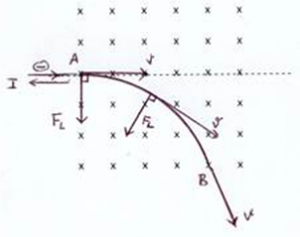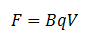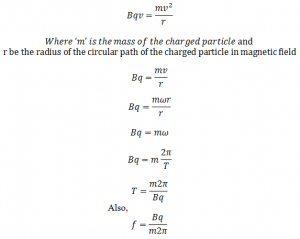# Motion Of A Charged Particle In Magnetic Fieldet us consider a charge particle having charge ‘q’ moves with velocity ‘v’ and enters into the region of magnetic field of strength ‘B’ as shown in fig.
According to Fleming left hand rule as the charge particle moves in the magnetic field, force experienced by the charge particle is:If the direction of charged particle is perpendicular to magnetic field,
In fig, a, b and c be the point where the velocity of charged particle remains constant i.e. ‘v’. If the magnetic field region is extended (constant magnetic field) the charged particle moves in the circular path. In this case the force experienced by the charge d particle is equal to that of the centripetal force,